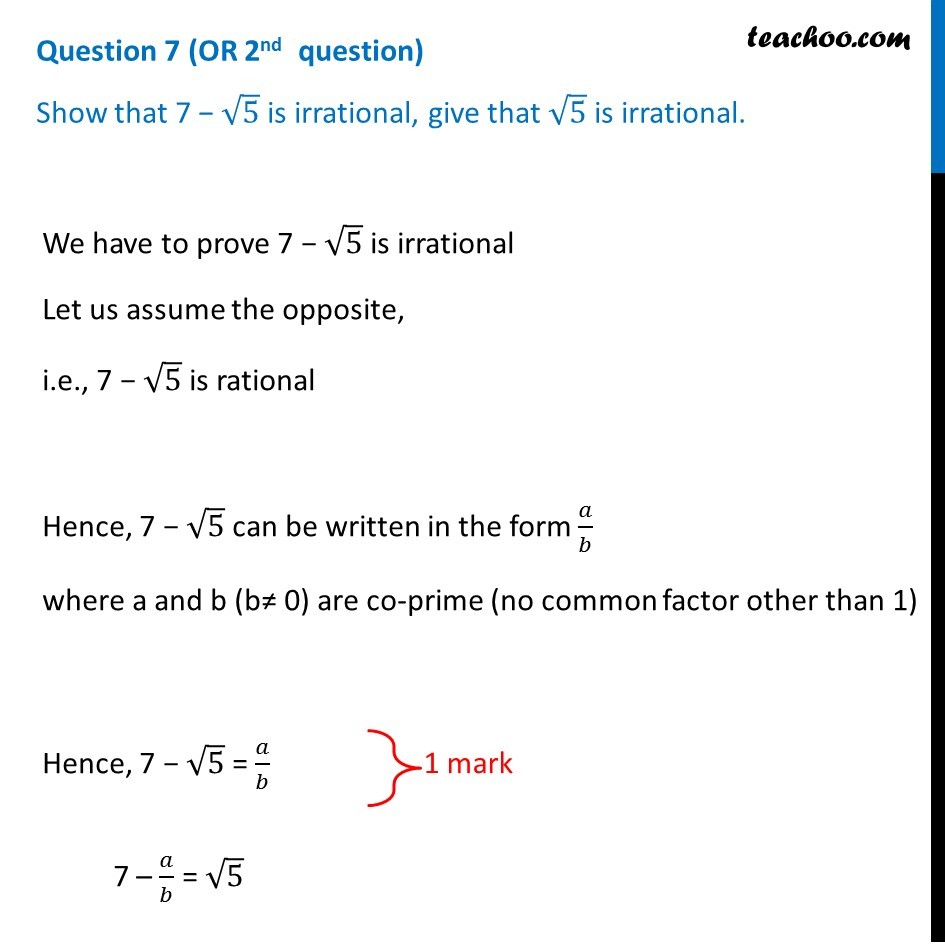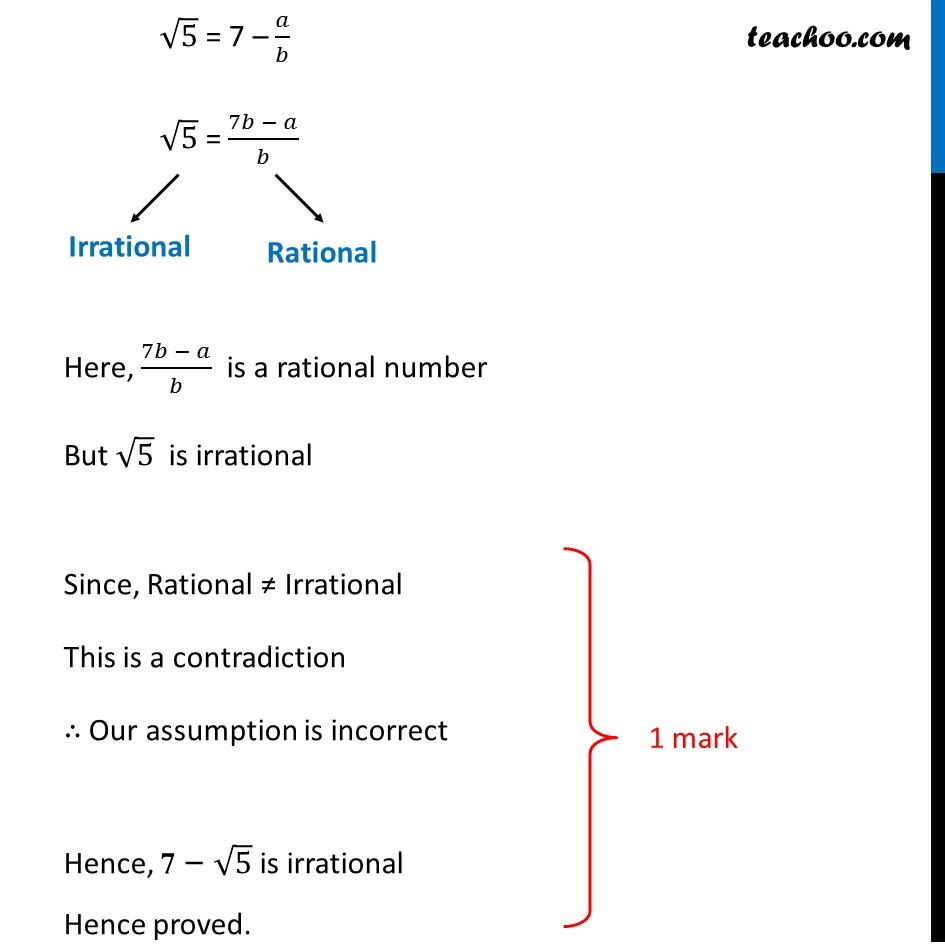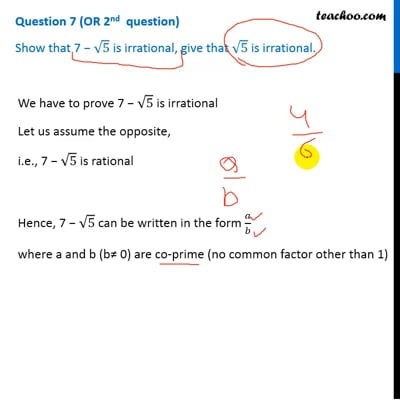CBSE Class 10 Sample Paper for 2019 Boards

Class 10
Solutions of Sample Papers for Class 10 Boards

Question 7 (OR 2 nd   question)

Show that 7 − √5 is irrational, give that √5 is irrational.This video is only available for Teachoo black users

### Transcript

Question 7 (OR 2nd question) Show that 7 − √5 is irrational, give that √5 is irrational. We have to prove 7 − √5 is irrational Let us assume the opposite, i.e., 7 − √5 is rational Hence, 7 − √5 can be written in the form 𝑎/𝑏 where a and b (b≠ 0) are co-prime (no common factor other than 1) Hence, 7 − √5 = 𝑎/𝑏 7 – 𝑎/𝑏 = √5 √5 = 7 – 𝑎/𝑏 √5 = (7𝑏 − 𝑎)/𝑏 Here, (7𝑏 − 𝑎)/𝑏 is a rational number But √5 is irrational Since, Rational ≠ Irrational This is a contradiction ∴ Our assumption is incorrect Hence, 7 − √5 is irrational Hence proved.